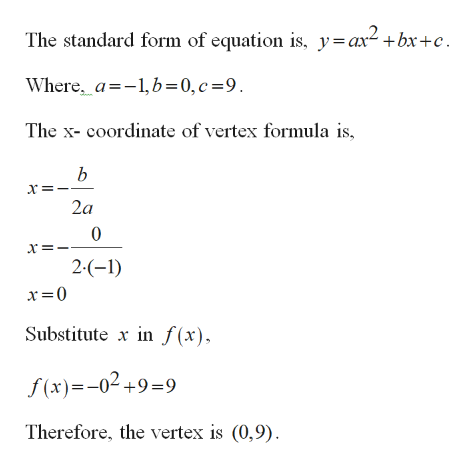# Graph, labeling the vertex and all x and y intercepts: f(x)=-x2+9

Question
4 views

Graph, labeling the vertex and all x and y intercepts: f(x)=-x2+9

check_circle

Step 1

The graph the function with labeling vertex, x- intercepts and y- intercepts.

Step 2

Find vertex for the function.help_outlineImage TranscriptioncloseThe standard form of equation is, y=ax2 +bx+c Where_a-1,b=0, c =9 The x- coordinate of vertex formula is b х: 2a 0 х 2-(-1) Substitute in f (x) f(x)-02+9=9 Therefore, the vertex is (0,9) fullscreen
Step 3

Find x-intercep...

### Want to see the full answer?

See Solution

#### Want to see this answer and more?

Solutions are written by subject experts who are available 24/7. Questions are typically answered within 1 hour.*

See Solution
*Response times may vary by subject and question.
Tagged in

### Algebra